Processing ......FreeComputerBooks.com Links to Free Computer, Mathematics, Technical Books all over the World

Topology Without Tears

• Title: Topology Without Tears
• Author(s) Sidney A. Morris
• Publisher: Internet
• Hardcover/Paperback: N/A
• eBook: PDF (727 pages) and Video Lectures
• Language: English, Arabic, Chinese, Greek, Korean, Persian, Russian, Spanish, Turkish
• ISBN-10: N/A
• ISBN-13: N/A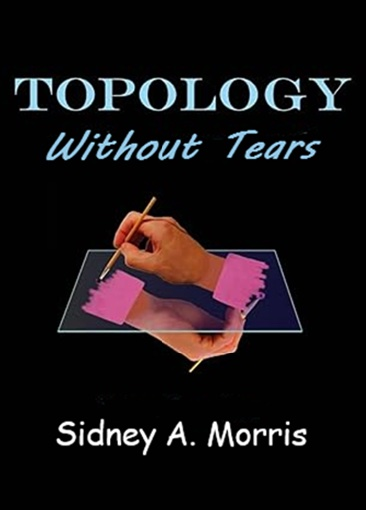Book Description

In mathematics, topology is concerned with the properties of a geometric object that are preserved under continuous deformations, such as stretching, twisting, crumpling, and bending; that is, without closing holes, opening holes, tearing, gluing, or passing through itself.

General topology is the branch of topology dealing with the basic set-theoretic definitions and constructions used in topology. It is the foundation of most other branches of topology, including algebraic topology, differential topology, geometric topology, and topological algebra.

Another name for general topology is point-set topology, is the main language for a broad range of mathematical disciplines, while algebraic topology offers as a powerful tool for studying problems in geometry and numerous other areas of mathematics.

The aim of this is to provide a thorough grouding of general topology. It offers an ideal introduction to the fundamentals of topology, and consists of a series of basic to intermediate lessons in topology.

• N/A
Reviews, Ratings, and Recommendations: Related Book Categories: Read and Download Links:Similar Books:
•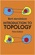Introduction to Topology (Bert Mendelson, et al.)

This concise book offers an ideal introduction to the fundamentals of topology. The book's principal aim is to provide a simple, thorough survey of elementary topics in the study of collections of objects, or sets, that possess a mathematical structure.

•Topology: A Categorical Approach (Tai-Danae Bradley, et al)

A graduate-level textbook that presents basic topology from the perspective of category theory. Many graduate students are familiar with the ideas of point-set topology and they are ready to learn something new about them.

•Topological Groups: Yesterday, Today, Tomorrow (Sidney A. Morris)

In 1900, David Hilbert asked whether each locally euclidean topological group admits a Lie group structure. This was the fifth of his famous 23 questions which foreshadowed much of the mathematical creativity of the twentieth century.

•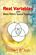Real Variables with Basic Metric Space Topology (Robert B. Ash)

Designed for a first course in real variables, this text presents the fundamentals for more advanced mathematical work, particularly in the areas of complex variables, measure theory, differential equations, functional analysis, and probability.

•Topology of Numbers (Allen Hatcher)

A textbook on elementary number theory from a geometric point of view, as opposed to the usual strictly algebraic approach. A fair amount of the book is devoted to studying Conway's topographs associated to quadratic forms in two variables.

•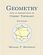Geometry with an Introduction to Cosmic Topology

The text uses Mobius transformations in the extended complex plane to define and investigate these three candidate geometries, thereby providing a natural setting in which to express results common to them all, as well as results that encapsulate key differences.

•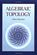Algebraic Topology (Allen Hatcher)

This introductory text is suitable for use in a course on the subject or for self-study, featuring broad coverage and a readable exposition, with many examples and exercises. The four main chapters present the basics: fundamental group and covering spaces, homology and cohomology, higher homotopy groups, and homotopy theory generally.

Book Categories
 :All CategoriesRecent BooksMiscellaneous BooksComputer LanguagesComputer ScienceData Science/DatabasesElectrical EngineeringJava and Java EE (J2EE)Linux and UnixMathematicsMicrosoft and .NETMobile ComputingNetworking and CommunicationsSoftware EngineeringSpecial TopicsWeb Programming
Other Categories